# Conjugate harmonic functions

(diff) ← Older revision | Latest revision (diff) | Newer revision → (diff)

harmonically-conjugate functions

A pair of real harmonic functionsandwhich are the real and imaginary parts of some analytic function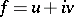of a complex variable. In the case of one complex variable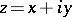, two harmonic functions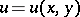andare conjugate in a domainof the complex planeif and only if they satisfy the Cauchy–Riemann equations in:(1)

The roles ofandin (1) are not symmetric:is a conjugate forbut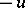, and not, is a conjugate for. Given a harmonic function, a local conjugate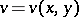and a local complete analytic functionare easily determined up to a constant term. This can be done, for example, using the Goursat formula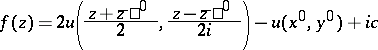(2)

in a neighbourhood of some point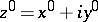in the domain of definition of.

In the case of several complex variables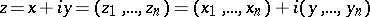,, the Cauchy–Riemann system becomes overdetermined(3)

It follows from (3) that for,can no longer be taken as an arbitrary harmonic function; it must belong to the subclass of pluriharmonic functions (cf. Pluriharmonic function). The conjugate pluriharmonic functioncan then be found using (2).

There are various analogues of conjugate harmonic functions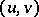involving a vector functionwhose components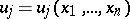are real functions of real variables. An example is a gradient system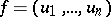satisfying the generalized system of Cauchy–Riemann equations(4)

which can also be written in abbreviated form: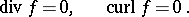If the conditions (4) hold in a domainof a Euclidean spacehomeomorphic to a ball, then there is a harmonic functiononsuch that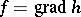. When, it turns out that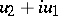is an analytic function of the variable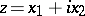. The behaviour of the solutions of (4) is in some respects similar to that of the Cauchy–Riemann system (1), for example in the study of boundary properties (see ).

How to Cite This Entry:
Conjugate harmonic functions. Encyclopedia of Mathematics. URL: http://encyclopediaofmath.org/index.php?title=Conjugate_harmonic_functions&oldid=17885
This article was adapted from an original article by E.D. Solomentsev (originator), which appeared in Encyclopedia of Mathematics - ISBN 1402006098. See original article